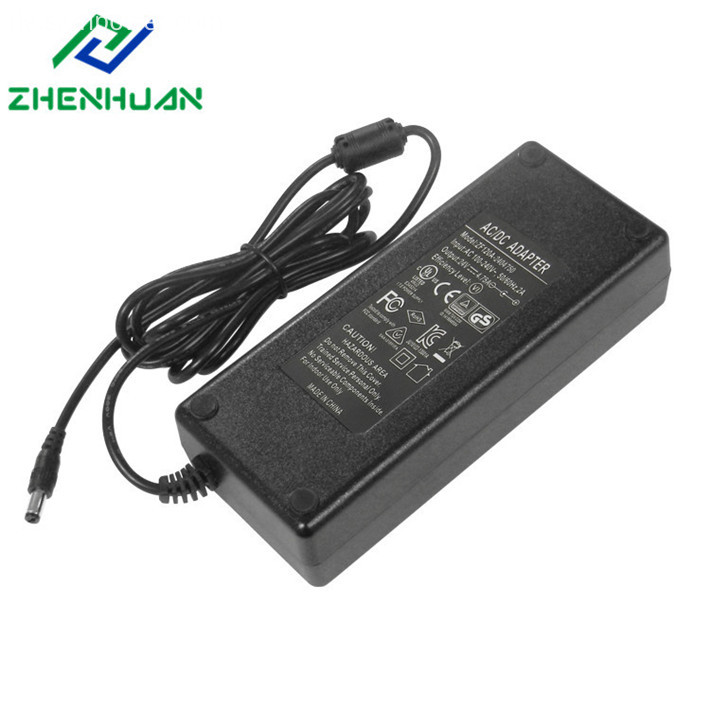Kontakt mit Lieferant? LieferantMs. Jane
Was kann ich für Sie tun?
Online ServiceKontaktieren jetzt

# Is the power adapter's no-load voltage exactly the same as the nominal voltage?

2019-07-16

Introduction: Is the power adapter's no-load voltage the same as the nominal voltage? The answer is: The actual no-load voltage of the common power adapter in the industry is not necessarily the same as the nominal voltage, while the civil situation, according to the needs of the application, is controlled from 0.1% to 5%. In fact, the smaller the error, the higher the consistency of the components.The actual no-load voltage of the common power adapter is not necessarily the same as the nominal voltage. Because the characteristics of the electronic components cannot be completely consistent, a certain amount of error is allowed, and the civil situation is controlled to be about 0.1% to 5% depending on the needs of the application. The smaller the error, the higher the requirement for the consistency of electronic components, the higher the cost in industrial production, and the more expensive the price.

The same nominal voltage power supply, the output current is different, can not be used in the same notebook. The basic principle is that a large nominal current source can replace a small nominal current source. Some friends have misunderstood and think that the power of the large nominal current will burn the notebook because the current is high. Actually how much current depends on the load under the same voltage, that is, the working condition of the notebook. When the notebook is running at a high load, the current is larger. When the notebook enters standby, the current is smaller, and the current is equal to the notebook. The voltage on the divided by the equivalent resistance of the notebook.

Followed by the nominal current value of the power supply. No matter what power supply has a certain internal resistance, when the power supply outputs current, it will generate a voltage drop internally, which is equal to the output current multiplied by the internal resistance of the power supply. Two things are caused, one is the generation of heat, which is equal to the square of the output current multiplied by the internal resistance, so the power supply is hot, and the other is that the output voltage becomes the nominal voltage minus the internal voltage drop, resulting in a decrease in the output voltage.

If the load resistance is too low, causing the output current to exceed the nominal current, two things usually occur. One is that the individual components burn due to heat exceeding the thermal capacity and cause the power supply to be damaged, and the other is the thermal design with margin, which is only reflected in The output voltage is further reduced. If it is reduced too much, the load may not work properly.

Liste verwandter Produkte

Zuhause

Phone

Skype

Anfrage